Vibroengineering PROCEDIA

Published: 02 April 2020

# Control the ride comfort of soil compactor with semi-active seat suspension and cab’s horizontal damper

Beiping Zhang1
Vanliem Nguyen2
Yong Wang3
1, 2, 3Hubei Polytechnic University, Huangshi City, China
Corresponding Author:
Vanliem Nguyen
Views 76

#### Abstract

To control the vibration of the driver’s seat in the vertical direction and the cab shaking angle in the pitching direction, a semi-active seat suspension and cab’s horizontal damper (SSS-HD) are proposed in this study. Based on the soil compactor dynamic model established in Matlab/Simulink software, the damping coefficients ${u}_{s}$ and ${u}_{x}$ of the SSS-HD are controlled via the PID-fuzzy control. The weighted root-mean-square acceleration responses of the vertical driver’s seat (${a}_{wzs}$) and pitching cab angle (${a}_{w\phi s}$) are selected as the evaluation indexes. The SSS-HD is then compared with the semi-active hydraulic mount (SHM) of the cab. The results show that the SSS-HD has a great effect in controlling the vibration of the driver’s driver in the vertical direction and cab shaking in comparison with the SHM of the cab under the same simulation conditions of the vehicle. In particular, the ${a}_{wzs}$ is improved by 27.9 % under the traveling condition and the ${a}_{w\phi s}$ is also lower 40.2 % under the compacting condition in comparison with the SHM.

## 1. Introduction

Under the vibration excitation of the drum/elasto-plastic soil in the compression condition and the deformed terrain with the poor surface roughness in the moving condition of the soil compactor, the driver’s seat vibration in the vertical direction and the cab shaking in the pitching direction were greatly affected [1-5]. Accordingly, the cab isolation system of the soil compactor had been constantly researched and improved in recent years. The traditional rubber mounts with their high stiffness and low damping coefficients which could only improve the vibration and noise at high-frequency range were mainly used for the cab isolation system of the soil compactor [3, 4, 6, 7]. This isolation system was then researched and replaced by using the hydraulic mounts [8, 9]. By using the hydraulic mounts, the cab’s ride comfort was greatly reduced in comparison with the traditional rubber mounts under the different operating conditions. However, the cab’s vibration response in both the vertical and pitching directions was still very high according to the standard of ISO 2631-1 , thus, it was uncomfortable for operators to control the soil compactors on the roadworks. To solve this problem, the semi-active hydraulic mounts (SHM) of the cab using the Fuzzy control and optimal PID-Fuzzy control were then researched and applied [9, 11, 12]. The results indicated that the cab shaking and the vertical vibration of the driver’s seat was better than the cab’s hydraulic mount without the control. However, these results were only obtained under the compacting condition of the vehicle. It was difficult to control both the vertical vibration of the driver’s seat and cab shaking. To control the cab shaking in the pitching direction, an auxiliary damper in the horizontal direction was installed on the cab , the result showed that the pitching cab angle ware significantly improved under the low-frequency range. Additionally, the driver’s seat suspension of the car and heavy truck using the semi-active suspension was also applied [13, 14], thus, the driver’s ride comfort was obviously improved. Based on the existed researches, the idea of this study is that a semi-active seat suspension and cab’s horizontal damper (SSS-HD) are proposed for the vehicle cab to further improve the vehicle’s ride comfort. A soil compactor dynamic model is established in Matlab/Simulink software, the damping coefficients ${u}_{s}$ and ${u}_{x}$ of the SSS-HD are then controlled via the PID-fuzzy control. The weighted root-mean-square acceleration responses of the vertical driver’s seat (${a}_{wzs}$) and pitching cab angle (${a}_{w\phi s}$) are selected as the evaluation indexes. The SSS-HD is then compared with the SHM of the cab to clarify the performance of SSS-HD.

## 2. Soil compactor dynamic model

Based on the soil compactor dynamic model with 7DOF , the cab isolation system equipped with the SHM is established in Fig. 1(a). The model of the cab isolation system in Fig. 1(a) is then replaced by using the passive hydraulic mount, a semi-active damping coefficient added to the cab in the horizontal direction and semi-active seat suspension are applied to control the vibration of the vertical driver’s seat and cab shaking, as described in Fig. 1(b), where ${Z}_{s}$, ${Z}_{c}$, and ${Z}_{b}$ are the displacements of the driver’s seat, cab, and rear vehicle frame in the vertical direction; ${\phi }_{c}$ and ${\phi }_{b}$ are the pitching angles of the cab and rear vehicle frame; ${m}_{s}$, ${m}_{c}$, and ${m}_{b}$ are the mass of the driver’s seat, cab, and rear vehicle frame; ${K}_{c1}$, ${K}_{c2}$ and ${C}_{c1}$, ${C}_{c2}$ are the passive stiffness and damping coefficients of the hydraulic mounts, while ${K}_{s}$ and ${C}_{s}$ are also the passive stiffness and damping coefficients of the driver’s seat suspension; ${u}_{c1}$ and ${u}_{c2}$ are the control damping coefficients of the SHM while ${u}_{s}$ and ${u}_{x}$ are the control damping coefficients of the SSS-HD; ${l}_{i}$ is the distances of the seat, cab, and vehicle, ($i=$ 1-5); and ${q}_{t}$ is the vibration excitation of the deformable terrain with the surface roughness.

Based on the dynamic model of the soil compactor in Fig. 1(a) and 1(b), their motion equations can be written in the matrix form as follows:

1
$M\stackrel{¨}{Z}+C\stackrel{˙}{Z}+KZ=F\left(u\right)+F\left(t\right),$

where $M$, $C$, and $K$ are the matrix of the mass, damping, and stiffness; $Z$ and $F\left(t\right)$ are the vectors of the displacement and exciting force; $F\left(u\right)$ is the SSS-DH or SHM’s control force vector.

Fig. 1The soil compactor dynamic model with the semi-active cab isolation systems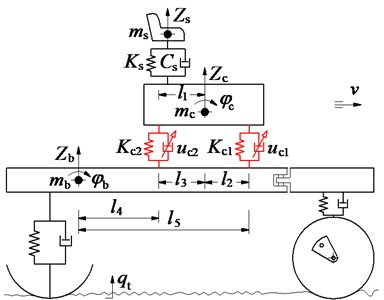a) The cab’s SHM model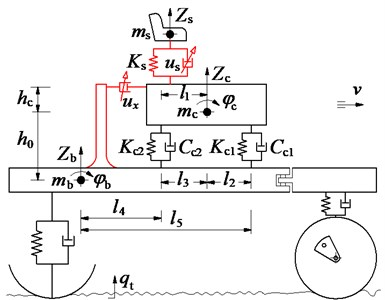b) The SSS-HD model of the seat and cab

## 3. Application of fuzzy control

To assess the performance of the SSS-HD for controlling the vibration of the driver’s seat and cab shaking, the control forces of both the SSS-HD and SHM are controlled via the semi-active damping coefficients of (${u}_{s}$ and ${u}_{x}$) and (${u}_{c1}$ and ${u}_{c2}$) which are computed based on the Fuzzy control and the vehicle dynamic model. The equations of the force and moment of the seat suspension (${F}_{s}$) and cab isolation system (${F}_{c1}$, ${F}_{c2}$, and ${M}_{cx}$) are described as follows:

2
${F}_{s}={K}_{s}\left({Z}_{c}+{l}_{1}{\stackrel{˙}{\phi }}_{c}-{Z}_{s}\right)+\left({C}_{s}+{u}_{s}\right)×\left({\stackrel{˙}{Z}}_{c}+{l}_{1}{\stackrel{˙}{\phi }}_{c}-{\stackrel{˙}{Z}}_{s}\right),$
3
${F}_{cn}={K}_{rn}\left({Z}_{b}-{l}_{m}{\phi }_{b}+\left(-1{\right)}^{n+1}{l}_{n+1}{\phi }_{c}\right)+\left({C}_{rn}+{u}_{rn}\right)\left({\stackrel{˙}{Z}}_{b}-{l}_{m}{\stackrel{˙}{\phi }}_{b}+\left(-1{\right)}^{n+1}{l}_{n+1}{\stackrel{˙}{\phi }}_{c}\right),$
4

where $n=$ 1, 2 then $m=$ 5, 4; ${M}_{cx}$ is the counter-torque which is created by the semi-active damping force ${F}_{cx}$ in the horizontal direction; ${h}_{0}$ and ${h}_{c}$ are the distances in the vertical direction between at the centre of gravity of the cab and rear vehicle frame; and between the centre of gravity of the cab and horizontal damper.

The control damping coefficients of the SSS-HD or SHM in Eqs. (2)-(4) are determined by:

5

In order to control the ${u}_{i}$ ($i=s$, $x$ or ${c}_{1}$, ${c}_{2}$), the various fuzzy controls need to design, and their design process is similar. Therefore, a Fuzzy control is established to control for all the semi-active damping coefficients of the SSS-HD or SHM as follows: Two variables of input are chosen by the deflection ${E}_{i}$ and its derivation $E{C}_{i}$, herein, $i=s$ and $x$, ${E}_{s}$ and ${E}_{x}$ are deflections of the seat suspension and horizontal damper of the SSS-HD; $i={c}_{1}$ and ${c}_{2}$, ${E}_{c1}$ and ${E}_{c2}$ are deflections of the front/rear cab isolation systems of the SHM. The semi-active damping coefficients ${u}_{i}$ is the output variables.

The linguistic variables of the input values are described as the positive big (pb), positive small (ps), zero (z), negative small (ns), and negative big (nb), while the output variables’ linguistic variables are described as the small (s), medium-small (ms), medium (m), medium-big (mb), and big (b). A Fuzzy set is used to perform the variables’ membership functions (MF), and the shape of the Triangular function with its value between 0 and 1 is then applied for the MF. The values of inputs are in a range of [–1, 1] while of outputs are in a range of [0, ${u}_{imax}$].

According to expertise experiences and designer’s knowledge, there have 25 rules of if-then of Fuzzy control are given in Table 1 and generally written as follows: ${R}_{im}$: If ${E}_{im}={D}_{i\alpha }$ and $E{C}_{im}={V}_{im}$ then ${u}_{im}={C}_{im}$, ($m=$ 25).

The inference system of the Fuzzy control chosen via the minimum function and the centroid method of Mamdani [9, 12, 16] is then applied in this study to control the system model.

## 4. Excitation vibration sources

In the actual operating condition of the soil compactor, two main excitation sources are the vibrator drum and the deformed terrain surface [1, 2, 4, 7]. This study uses the existed result of the model of the drum/elasto-plastic soil interaction in Ref.  to determine the excitation force of the drum, and model of the wheel-deformed terrain contact in Ref. [17, 18] to determine the force of the wheel under the surface roughness of the deformed terrain.

The time history of the poor terrain surface at the vehicle speed 5 km/h is then simulated under the condition of the vehicle traveling, and plotted as in Fig. 2.

## 5. Result and discussion

To evaluate the performance of the SSS-HD for reducing the vibration of the vertical driver’s seat and cab shaking, the weighted root-mean-square acceleration responses of the vertical driver’s seat (${a}_{wzs}$) and pitching cab angle (${a}_{w\phi s}$) given in Refs. [10, 4] are selected as the two evaluation indexes. Also, based on the control rules in Table 1 and the lumped parameters of the vehicle in Table 2, Matlab R2015b/Simulink software is applied to simulate to evaluate the control performance under two operating conditions of the vehicle as follows:

Fig. 2Time history of the terrain surface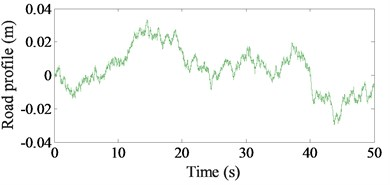Table 1The control rules of Fuzzy control

 ${u}_{i}$ ${E}_{i}$ nb ns z ps pb $E{C}_{i}$ nb b mb m ms z ns mb mb m z ms z m m z m m ps ms z m mb mb pb z ms m mb b

Table 2Lumped parameters of the soil compactor cab with SSS-HD and SHM

 Parameter Value Parameter Value Parameter Value Parameter Value ${m}_{s}$ / kg 85 ${h}_{0}$ / m 0.1 ${l}_{4}$ / m 0.136 ${K}_{c2}$ / Nm-1 1.2×105 ${m}_{c}$ / kg 891 ${l}_{1}$ / m 0.383 ${l}_{5}$ / m 0.76 ${C}_{s}$ / Ns m-1 1.2×102 ${m}_{b}$ / kg 4464 ${l}_{2}$ / m 0.1 ${K}_{s}$ / Nm-1 1.2×104 ${C}_{c1}$ / Ns m-1 20×103 ${h}_{c}$ / m 0.4 ${l}_{3}$ / m 0.524 ${K}_{c1}$ / Nm-1 9.1×105 ${C}_{c2}$ / Ns m-1 4.5×103

### 5.1. Control performance on deformed terrain

The existed researches show that the driver’s seat ride comfort and cab shaking are strongly affected when the vehicle travels on the poor terrain surface into the roadworks [3, 9]. Thus, assuming that the vehicle travels on the poor terrain surface of deformed terrain (as given in Fig. 2) with a speed of 5 km/h to evaluate the performance of the SSS-HD. The acceleration results of the vertical driver’s seat and pitching cab angle are then plotted in Fig. 3(a) and 3(b).

Fig. 3The result of the acceleration responses under the traveling condition of the vehicle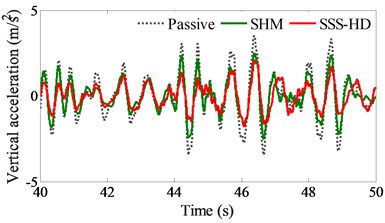a) The vertical acceleration of the driver’s seat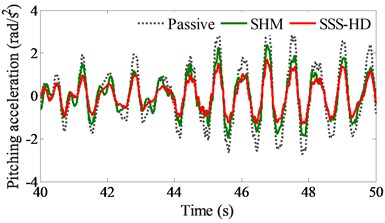b) The pitching acceleration of the cab

Table 3The calculated results of the awzs and awφc with SSS-HD and SHM

 Simulation conditions The traveling condition The compacting condition Control ${a}_{wzs}$ (m/s2) ${a}_{w\phi c}$(rad/s2) ${a}_{wzs}$ (m/s2) ${a}_{w\phi c}$ (rad/s2) Passive 1.0058 0.9319 0.5910 0.2660 SHM 0.6972 0.6103 0.3939 0.2224 SSS-HD 0.5022 0.4843 0.3283 0.1330 Comparison between SSS-HD & SHM 27.9 % 20.6 % 16.6 % 40.2 %

With using the SSS-HD, the simulation result shows that both the acceleration responses of the vertical driver’s seat and pitching cab angle are strongly reduced in comparison with the SHM. The driver’s seat acceleration is greatly decreased due to direct control of the damping coefficient of the seat suspension while cab shaking is also strongly reduced due to the counter-torque ${M}_{cx}={F}_{cx}×{h}_{c}$ which is created by the semi-active damping force ${F}_{cx}$ in the horizontal direction and distance ${h}_{c}$ between the centre of gravity of the cab and horizontal damper.

Also, the results of the reduced RMS values of the ${a}_{wzs}$ and ${a}_{w\phi c}$ are also clearly reflected in Table 3. Both the ${a}_{wzs}$ and ${a}_{w\phi c}$ values with the SSS-HD are reduced by 27.9 % and 20.6 % in comparison with the SHM, especially the driver’s seat acceleration, therefore, it can be concluded that the vehicle’s ride comfort and cab shaking with the SSS-HD are better than the SHM under the same simulation and control condition.

### 5.2. Control performance on elasto-plastic soil

In the compacting condition, the researches indicate that the cab shaking is strongly influenced on the sandy ground with its low-density of elasto-plastic soil at the excitation 28 Hz of the drum [3, 4]. Thus, the performance of the SSS-HD is also evaluated under the same compacting condition of the vehicle at the excitation 28 Hz of the drum with the vehicle speed of 3 km/h, and the lumped parameters of the sandy terrain with low-density are used in Ref. . The acceleration results of the vertical driver’s seat and pitching cab angle are shown in Fig. 4.

Similarly, to the condition of the vehicle traveling, the simulation results in both Fig. 4(a) and 4(b) also indicate that the acceleration responses of the vertical driver’s seat and pitching cab angle with the SSS-HD are significantly decreased comparable with the SHM. This good result is also due to the impact of both semi-active damping forces created by both the ${u}_{s}$ and ${u}_{x}$ of the seat suspension and horizontal damper of the cab. Besides, the results are also assessed via the RMS values of both the ${a}_{wzs}$ and ${a}_{w\phi c}$ in the same Table 3. Comparing with the SHM, the ${a}_{wzs}$ and ${a}_{w\phi c}$ are decreased by 16.6 % and 40.2 % with the SSS-HD, particularly the pitching cab angle. Therefore, based on the above analysis results of the SSS-HD under various operating conditions of the soil compactors, it can be concluded that the SSS-HD has a clear effect on the cab shaking control and the driver’s seat ride comfort in comparison with the SHM.

Fig. 5The result of the acceleration responses under the compacting condition of the vehicle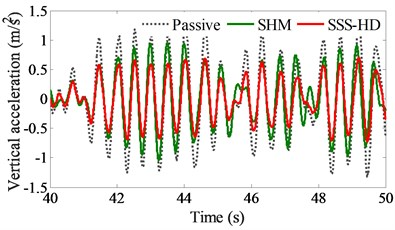a) The vertical acceleration of the driver’s seat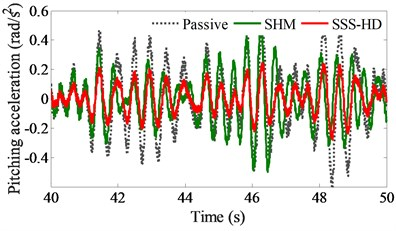b) The pitching acceleration of the cab

## 6. Conclusions

In this paper, an auxiliary damper is added on the cab in the horizontal direction, the damping coefficients of the horizontal damper ${u}_{x}$ and seat suspension ${u}_{s}$ are then controlled to improve the cab shaking and driver’s seat ride comfort. The main conclusions can be summarized as follows:

1) Under various operating conditions of the soil compactors, both the ${a}_{wzs}$ and ${a}_{w\phi c}$ with the SSS-HD are greatly reduced in comparison with the SHM, especially, the vertical driver’s seat vibration is reduced by 27.9 % under the traveling condition and the cab shaking is reduced by 40.2 % under the compacting condition.

2) In the existed researches, the SHM of the cab used by the optimal control method significantly improves the ride comfort of the vehicle [9, 15].

However, the vertical vibration of the driver’s seat and cab shaking is quite high. By using the SSS-HD, both the cab shaking and driver’s seat ride comfort are greatly reduced under the same simulation and control conditions. The results of this study can be referenced to improve the cab isolation systems of the soil compactors.

#### Acknowledgements

This study is supported by Hubei Province Education and Research Projects (No. 2018452).